# Visualizing Data with Pandas and Matplotlib¶

### David Backus¶

We illustrate three approaches to graphing data with Python's Matplotlib package:

• Approach #1: Apply a plot() method to a dataframe
• Approach #2: Use the plot(x,y) function
• Approach #3: Create a figure object and apply methods to it

The last one is the least intuitive but also the most useful. We work up to it gradually. This book chapter covers the same material with more words and fewer pictures.

This IPython notebook was created by Dave Backus for the NYU Stern course Data Bootcamp.

## Preliminaries¶

### Jupyter¶

Look around, what do you see? Check out the menubar at the top: File, Edit, etc. Also the toolbar below it. Click on Help -> User Interface Tour for a tour of the landscape.

The cells below come in two forms. Those labeled Code (see the menu in the toolbar) are Python code. Those labeled Markdown are text.

### Markdown¶

Markdown is a user-friendly language for text formatting. You can see how it works by clicking on any of the Markdown cells and looking at the raw text that underlies it. In addition to just plain text, we'll use three things a lot:

• Bold and italics. The raw text **bold** displays as bold. The raw text *italics* displays as italics.
• Bullet lists. If we want a list of items marked by bullets, we start with a blank line and mark each item with an asterisk on a new line. Double click on this cell for an example.
• Headings. We create section headings by putting a hash in front of the text. # Heading gives us a large heading. Two hashes a smaller heading, three hashes smaller still, up to four hashes. In this cell there's a two-hash heading at the top.

Exercise. Click on the blank cell below. Note that it's labeled Markdown in the menubar. Add a heading and some text. Execute the cell by either (i) clicking on the "run cell" button in the toolbar or (ii) clicking on "Cell" in the menubar and choosing Run.

### Import packages¶

In :
import sys                             # system module
import pandas as pd                    # data package
import matplotlib as mpl               # graphics package
import datetime as dt                  # date and time module

# check versions (overkill, but why not?)
print('Python version:', sys.version)
print('Pandas version: ', pd.__version__)
print('Matplotlib version: ', mpl.__version__)
print('Today: ', dt.date.today())

Python version: 3.5.1 |Anaconda 2.4.1 (64-bit)| (default, Dec  7 2015, 11:16:01)
[GCC 4.4.7 20120313 (Red Hat 4.4.7-1)]
Pandas version:  0.17.1
Matplotlib version:  1.5.0
Today:  2016-01-13


Comment. When you run the code cell above, its output appears below it.

In :
# This is an IPython command.  It puts plots here in the notebook, rather than  a separate window.
%matplotlib inline


### Create dataframes to play with¶

• US GDP and consumption
• World Bank GDP per capita for several countries
• Fama-French equity returns
In :
# US GDP and consumption
gdp  = [13271.1, 13773.5, 14234.2, 14613.8, 14873.7, 14830.4, 14418.7,
14783.8, 15020.6, 15369.2, 15710.3]
pce  = [8867.6, 9208.2, 9531.8, 9821.7, 10041.6, 10007.2, 9847.0, 10036.3,
10263.5, 10449.7, 10699.7]
year = list(range(2003,2014))        # use range for years 2003-2013

# create dataframe from dictionary
us = pd.DataFrame({'gdp': gdp, 'pce': pce}, index=year)

          gdp     pce
2003  13271.1  8867.6
2004  13773.5  9208.2
2005  14234.2  9531.8

In :
# GDP per capita (World Bank data, 2013, thousands of USD)
code    = ['USA', 'FRA', 'JPN', 'CHN', 'IND', 'BRA', 'MEX']
country = ['United States', 'France', 'Japan', 'China', 'India',
'Brazil', 'Mexico']
gdppc   = [53.1, 36.9, 36.3, 11.9, 5.4, 15.0, 16.5]

wbdf = pd.DataFrame({'gdppc': gdppc, 'country': country}, index=code)
wbdf

Out:
country gdppc
USA United States 53.1
FRA France 36.9
JPN Japan 36.3
CHN China 11.9
IND India 5.4
BRA Brazil 15.0
MEX Mexico 16.5

Comment. In the previous cell, we used the print() function to produce output. Here we just put the name of the dataframe. The latter displays the dataframe -- and formats it nicely -- if it's the last line in the cell.

In :
# Fama-French

# read annual data from website and rename variables
ff.columns = ['xsm', 'smb', 'hml', 'rf']
ff['rm'] = ff['xsm'] + ff['rf']
ff = ff[['rm', 'rf']]     # extract rm and rf (return on market, riskfree rate, percent)

Out:
rm rf
Date
2010 17.49 0.12
2011 0.48 0.04
2012 16.34 0.06
2013 35.21 0.02
2014 11.72 0.02

Comment. The warning in pink tells us that the Pandas DataReader will be spun off into a separate package in the near future.

Exercise. What kind of object is wb? How would you access its column and row labels? What are they?

In [ ]:


In :
# This is an IPython command:  it puts plots here in the notebook, rather than a separate window.
%matplotlib inline


## Digression: Graphing in Excel¶

Remind yourself that we need to choose:

• Data. Typically a block of cells in a spreadsheet.
• Chart type. Lines, bars, scatter, or something else.
• x and y variables. What is the x axis? What is y?

We'll see the same in Matplotlib.

## Approach #1: Apply plot() method to dataframe¶

Good simple approach, we use it a lot. It comes with some useful defaults:

• Data. The whole dataframe.
• Chart type. We have options for lines, bars, or other things.
• x and y variables. By default, the x variable is the dataframe's index and the y variables are all the columns of the dataframe.

All of these things can be changed, but this is the starting point.

Let's do some examples, see how they work.

### US GDP and consumption¶

In :
# try this with US GDP
us.plot()

Out:
<matplotlib.axes._subplots.AxesSubplot at 0x7fdcd1a27470>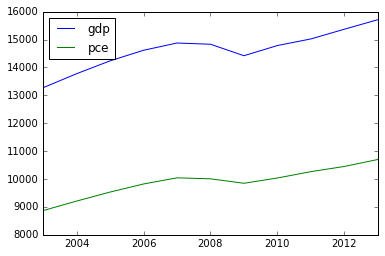In :
# do GDP alone
us['gdp'].plot()

Out:
<matplotlib.axes._subplots.AxesSubplot at 0x7fdcd25355f8>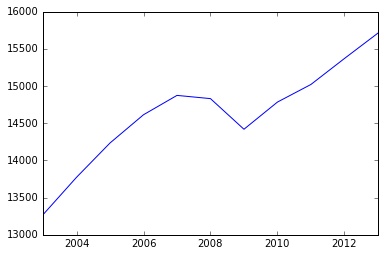In :
# bar chart
us.plot(kind='bar')

Out:
<matplotlib.axes._subplots.AxesSubplot at 0x7fdcd225f208>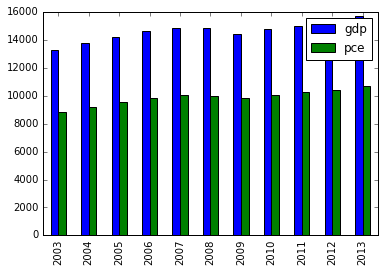Exercise. Show that we get the output from us.plot.bar().

In :
us.plot

Out:
<pandas.tools.plotting.FramePlotMethods object at 0x7fdcd1c72c50>
In :
# scatter plot
# we need to be explicit about the x and y variables: x = 'gdp', y = 'pce'
us.plot.scatter('gdp', 'pce')

Out:
<matplotlib.axes._subplots.AxesSubplot at 0x7fdcd1cc8fd0>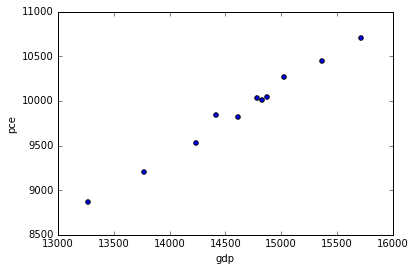Comment. We can get help in IPython by adding a question mark after a function or method.

Exercise. How can you get help for us.plot()? Try it and see.

Exercise. Add each of these arguments/parameters to us.plot()in the code cell below and describe what they do:

• kind='area'
• subplots=True
• sharey=True
• figsize=(3,6)
• xlim=(0,16000)
In [ ]:



### Fama-French asset returns¶

In :
# now try a few things with the Fama-French data
ff.plot()

Out:
<matplotlib.axes._subplots.AxesSubplot at 0x7fdcd18ac4a8>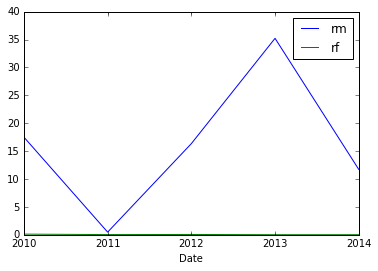Exercise. We can dress up the plots using the arguments of the plot() function. Try adding, one at a time, the arguments title='Fama-French returns', grid=True, and legend=False. What does the documentation say about them? What do they do?

In :
ff.plot()

Out:
<matplotlib.axes._subplots.AxesSubplot at 0x7fdcd188d278>Exercise. What do each of the arguments do in the code below?

In :
ff.plot(kind='hist', bins=20, subplots=True)

Out:
array([<matplotlib.axes._subplots.AxesSubplot object at 0x7fdcd180c630>,
<matplotlib.axes._subplots.AxesSubplot object at 0x7fdcd172c080>], dtype=object)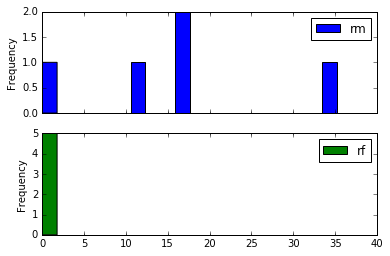Exercise. What do you see here? How do the returns differ?

In :
ff.plot(kind='kde', subplots=True, sharex=True)    # smoothed histogram ("kernel density estimate")

Out:
array([<matplotlib.axes._subplots.AxesSubplot object at 0x7fdcd16aa400>,
<matplotlib.axes._subplots.AxesSubplot object at 0x7fdcd1599be0>], dtype=object)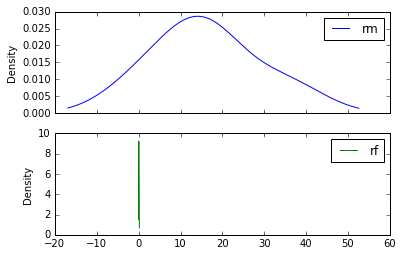### World Bank data¶

Exercise. Use the World Bank dataframe wbdf to create a bar chart of GDP per capita. Bonus points: Create a horizontal bar chart.

In [ ]:



## Approach #2: the plot(x,y) function¶

Here we plot variable y against variable x. This comes closest to what we would do in Excel: identify a dataset, a plot type, and the x and y variables, then press play.

In :
# import pyplot module of Matplotlib
import matplotlib.pyplot as plt

In :
plt.plot(us.index, us['gdp'])

Out:
[<matplotlib.lines.Line2D at 0x7fdcd150d2b0>]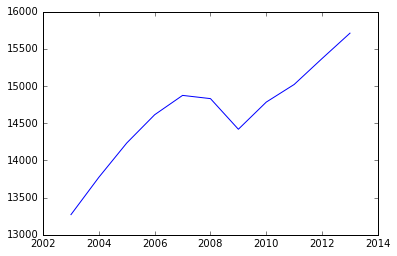Exercise. What is the x variable here? The y variable?

In :
# we can do two lines together
plt.plot(us.index, us['gdp'])
plt.plot(us.index, us['pce'])

Out:
[<matplotlib.lines.Line2D at 0x7fdcd1476550>]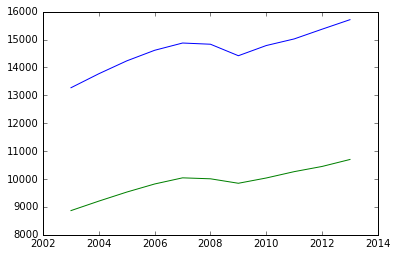In :
# or a bar chart
plt.bar(us.index, us['gdp'], align='center')

Out:
<Container object of 11 artists>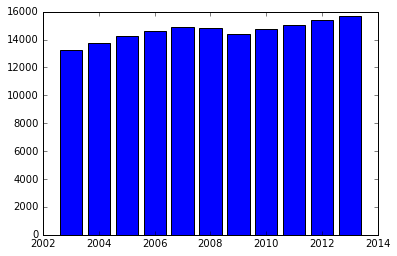Exercise. Experiment with

plt.bar(us.index, us['gdp'],
align='center',
alpha=0.65,
color='red',
edgecolor='green')


Play with the arguments one by one to see what they do. Or use plt.bar? to look them up. Add comments to remind yourself. Bonus points: Can you make this graph even uglier?

In [ ]:


In :
# we can also add things to plots
plt.plot(us.index, us['gdp'])
plt.plot(us.index, us['pce'])

plt.title('US GDP', fontsize=14, loc='left') # add title
plt.ylabel('Billions of 2009 USD')           # y axis label
plt.xlim(2002.5, 2013.5)                     # shrink x axis limits
plt.tick_params(labelcolor='red')            # change tick labels to red
plt.legend(['GDP', 'Consumption'])           # more descriptive variable names

Out:
<matplotlib.legend.Legend at 0x7fdcd13d6f60>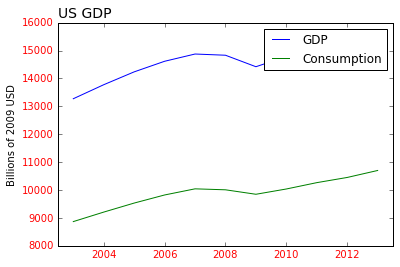Comment. All of these statements must be in the same cell for this to work.

Comment. This is overkill -- it looks horrible -- but it makes the point that we control everything in the plot. We recommend you do very little of this until you're more comfortable with the basics.

Exercise. Add a plt.ylim() statement to make the y axis start at zero, as it did in the bar charts. Bonus points: Change the color to magenta and the linewidth to 2. Hint: Use plt.ylim? and plt.plot? to get the documentation.

In [ ]:



Exercise. Create a line plot for the Fama-French dataframe ff that includes both returns. Bonus points: Add a title and label the y axis.

In [ ]:



## Approach #3: Create figure objects and apply methods¶

This approach is the most foreign to beginners, but now that we’re used to it we like it a lot. We either use it on its own, or adapt its functionality to the dataframe plot methods we saw in Approach #1. The idea is to generate an object – two objects, in fact – and apply methods to them to produce the various elements of a graph: the data, their axes, their labels, and so on.

In :
# create fig and ax objects
fig, ax = plt.subplots()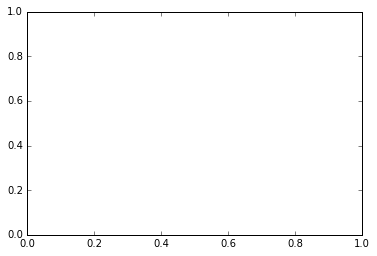Exercise. What do we have here? What type are fig and ax?

In [ ]:



We say fig is a figure object and ax is an axis object. This means:

• fig is a blank canvas for creating a figure.
• ax is everything in it: axes, labels, lines or bars, and so on.

Exercise. Use tab completion to see what methods are available for fig and ax. What do you see? Do you feel like screaming?

In [ ]:


In :
# let's try that again, this time with content
# create objects
fig, ax = plt.subplots()

# add things by applying methods to ax
ax.plot(us.index, us['gdp'], linewidth=2, color='magenta')
ax.set_title('US GDP', fontsize=14, loc='left')
ax.set_ylabel('Billions of USD')
ax.set_xticks([2004, 2008, 2012])
ax.grid(True)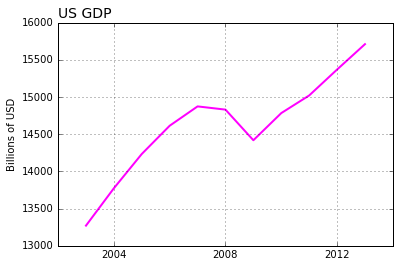Comment. All of these statements must be in the same cell.

In :
# a figure method: save figure as a pdf
fig.savefig('us_gdp.pdf')


Exercise. Use figure and axis objects to create a bar chart of variable rm in the ff dataframe.

In [ ]:



### Multiple subplots¶

Same idea, but we create a multidimensional ax and apply methods to each component. Here we redo the plots of US GDP and consumption.

In :
# this creates a 2-dimensional ax
fig, ax = plt.subplots(nrows=2, ncols=1, sharex=True)
print('Object ax has dimension', len(ax))

Object ax has dimension 2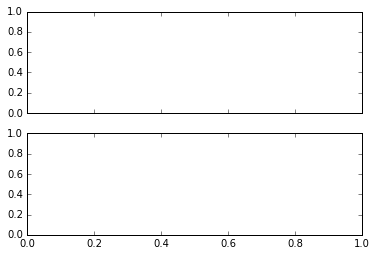In :
# now add some content
fig, ax = plt.subplots(nrows=2, ncols=1, sharex=True)

ax.plot(us.index, us['gdp'], color='green')   # first plot
ax.plot(us.index, us['pce'], color='red')     # second plot

Out:
[<matplotlib.lines.Line2D at 0x7fdcd11854a8>]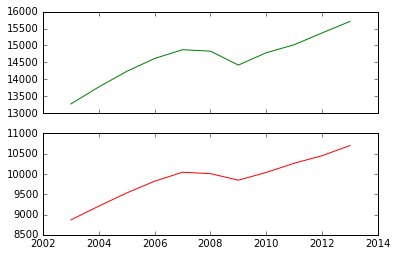### Approach #1 revisited¶

In Approach #1, we applied plot() and related methods to a dataframe. We also used arguments to fix up the graph, but that got complicated pretty quickly.

Here we combine Approaches 1 and 3. If we check the documentation of df.plot() we see that it "returns" an axis object. We can assign it to a variable and then apply methods to make the figure more compelling.

In :
# grab the axis
ax = us.plot()In :
# grab it and apply methods
ax = us.plot()
ax.set_title('US GDP and Consumption', fontsize=14, loc='left')
ax.set_ylabel('Billions of 2013 USD')
ax.legend(loc='center right')

Out:
<matplotlib.legend.Legend at 0x7fdcd11166d8>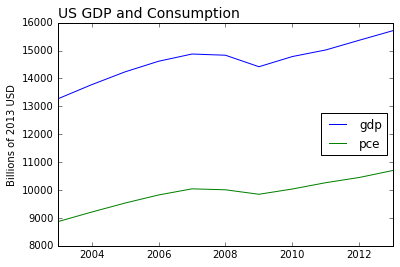Comment. If we want the figure object for this plot, we apply a method to the axis object ax:

fig = ax.get_figure()


That's not something we'll do often, but it completes the connection between Approaches #1 and #3.

In [ ]:



## Quick review of the bidding¶

Take a deep breath. We've covered a lot of ground, let's take stock.

We looked at three ways to use Matplotlib:

• Approach #1: apply plot method to dataframe
• Approach #2: use plot(x,y) function
• Approach #3: create fig, ax objects, apply plot methods to them

Same result, different syntax. This is what each of them looks like applied to US GDP:

us['gdp'].plot()                   # Approach #1

plt.plot(us.index, us['gdp'])      # Approach #2

fig, ax = plt.subplots()           # Approach #3
ax.plot(us.index, us['gdp'])


## Examples¶

We conclude with examples that take the data from the previous chapter and make better graphs with it.

### Student test scores (PISA)¶

The international test scores often used to compare quality of education across countries.

In :
# data input
import pandas as pd
url = 'http://dx.doi.org/10.1787/888932937035'
skiprows=18,     # skip the first 18 rows
skipfooter=7,    # skip the last 7
parse_cols=[0,1,9,13], # select columns
index_col=0,     # set index = first column
)
pisa = pisa.dropna()                          # drop blank lines
pisa.columns = ['Math', 'Reading', 'Science'] # simplify variable names

In :
# simple plot
pisa['Math'].plot(kind='barh')

Out:
<matplotlib.axes._subplots.AxesSubplot at 0x7fdcd103c4e0>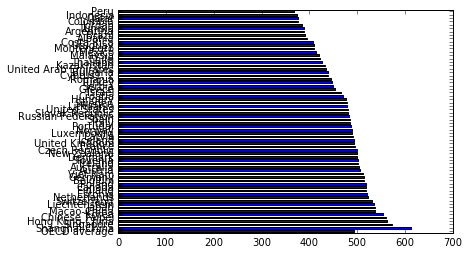Comment. Yikes! That's horrible! What can we do about it?

Let's make the figure taller. The figsize argument has the form (width, height). The default is (6, 4). We want a tall figure, so we need to increase the height setting.

In :
# make the plot taller
ax = pisa['Math'].plot(kind='barh', figsize=(4,13))  # note figsize
ax.set_title('PISA Math Score', loc='left')

Out:
<matplotlib.text.Text at 0x7fdcd0e1b160>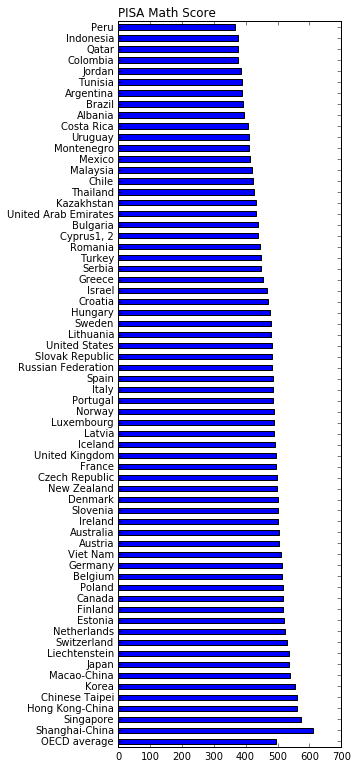Comment. What if we wanted to make the US bar red? This is ridiculously complicated, but we used our Google fu and found a solution. Remember: The solution to many problems is Google fu + patience.

In :
ax = pisa['Math'].plot(kind='barh', figsize=(4,13))
ax.set_title('PISA Math Score', loc='left')
ax.get_children().set_color('r')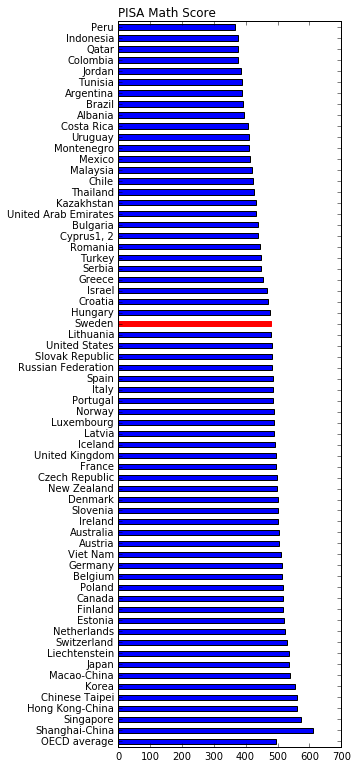Exercise. Create the same graph for the Reading score.

In [ ]:



### World Bank data¶

We'll use World Bank data for GDP, GDP per capita, and life expectancy to produce a few graphs and illsutrate some methods we haven't seen yet.

• Bar charts of GDP and GDP per capita
• Scatter plot (bubble plot) of life expectancy v GDP per capita
In :
# load packages (redundancy is ok)
import pandas as pd                   # data management tools
from pandas_datareader import data, wb # World Bank api
import matplotlib.pyplot as plt       # plotting tools

# variable list (GDP, GDP per capita, life expectancy)
var = ['NY.GDP.PCAP.PP.KD', 'NY.GDP.MKTP.PP.KD', 'SP.DYN.LE00.IN']
# country list (ISO codes)
iso = ['USA', 'FRA', 'JPN', 'CHN', 'IND', 'BRA', 'MEX']
year = 2013

# get data from World Bank

# massage data
df = df.reset_index(level='year', drop=True)
df.columns = ['gdppc', 'gdp', 'life'] # rename variables
df['pop']  = df['gdp']/df['gdppc']    # population
df['gdp'] = df['gdp']/10**12          # convert to trillions
df['gdppc'] = df['gdppc']/10**3       # convert to thousands
df['order'] = [5, 3, 1, 4, 2, 6, 0]   # reorder countries
df = df.sort_values(by='order', ascending=False)
df

Out:
gdppc gdp life pop order
country
Mexico 16.140664 1.997247 76.532659 1.237401e+08 6
Brazil 15.222320 3.109302 74.122439 2.042594e+08 5
India 5.131826 6.566166 67.660415 1.279499e+09 4
China 11.805087 16.023988 75.353024 1.357380e+09 3
Japan 35.614310 4.535077 83.331951 1.273386e+08 2
France 37.306283 2.459435 81.968293 6.592550e+07 1
United States 51.281583 16.230494 78.841463 3.164975e+08 0
In :
# GDP bar chart
ax = df['gdp'].plot(kind='barh', alpha=0.5)
ax.set_title('GDP', loc='left', fontsize=14)
ax.set_xlabel('Trillions of US Dollars')
ax.set_ylabel('')

Out:
<matplotlib.text.Text at 0x7fdcd132d208>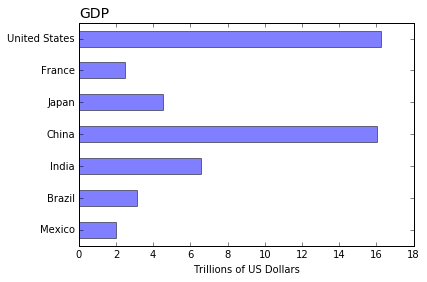In :
# ditto for GDP per capita (per person)
ax = df['gdppc'].plot(kind='barh', color='m', alpha=0.5)
ax.set_title('GDP Per Capita', loc='left', fontsize=14)
ax.set_xlabel('Thousands of US Dollars')
ax.set_ylabel('')

Out:
<matplotlib.text.Text at 0x7fdcd0c35128>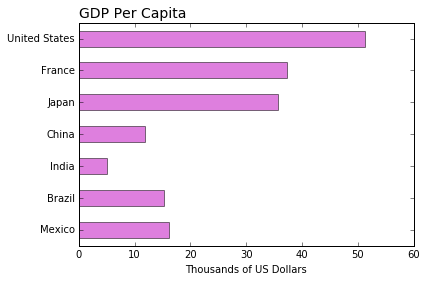And just because it's fun, here's an example of Tufte-like axes from Matplotlib examples. If you want to do this yourself, copy the last six line and prepare yourself to sink some time into it.

In :
# ditto for GDP per capita (per person)
ax = df['gdppc'].plot(kind='barh', color='b', alpha=0.5)
ax.set_title('GDP Per Capita', loc='left', fontsize=14)
ax.set_xlabel('Thousands of US Dollars')
ax.set_ylabel('')

# Tufte-like axes
ax.spines['left'].set_position(('outward', 10))
ax.spines['bottom'].set_position(('outward', 10))
ax.spines['right'].set_visible(False)
ax.spines['top'].set_visible(False)
ax.yaxis.set_ticks_position('left')
ax.xaxis.set_ticks_position('bottom')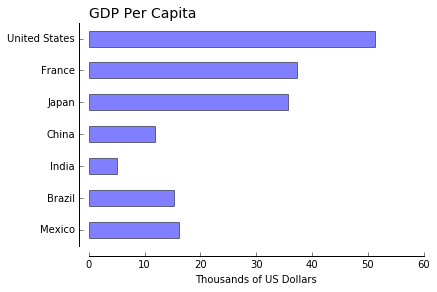In :
# scatterplot of life expectancy vs gdp per capita
plt.scatter(df['gdppc'], df['life'],    # x,y variables
s=df['pop']/10**6,          # size of bubbles
alpha=0.5)
plt.title('Life expectancy vs. GDP per capita', loc='left', fontsize=14)
plt.xlabel('GDP Per Capita')
plt.ylabel('Life Expectancy')
plt.text(58, 66, 'Bubble size represents population', horizontalalignment='right',)

Out:
<matplotlib.text.Text at 0x7fdcd0c098d0>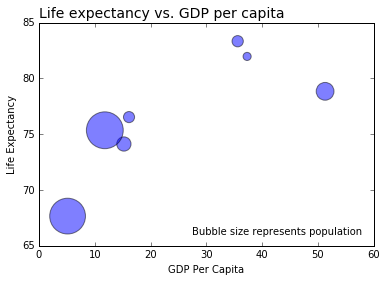## Styles (optional)¶

Graph settings you might like.

In :
ax = df['gdp'].plot(kind='barh', alpha=0.5)
ax.set_title('GDP', loc='left', fontsize=14)
ax.set_xlabel('Trillions of US Dollars')
ax.set_ylabel('')

Out:
<matplotlib.text.Text at 0x7fdcd0bbdb00>Exercise. Create the same graph with this statement at the top:

plt.style.use('fivethirtyeight')


(Once we execute this statement, it stays executed.)

Comment. We can get a list of files from plt.style.available.

In :
plt.style.available

Out:
['seaborn-dark',
'seaborn-notebook',
'seaborn-poster',
'seaborn-dark-palette',
'classic',
'seaborn-whitegrid',
'seaborn-deep',
'fivethirtyeight',
'grayscale',
'seaborn-muted',
'seaborn-white',
'seaborn-talk',
'seaborn-paper',
'seaborn-bright',
'seaborn-pastel',
'seaborn-darkgrid',
'seaborn-ticks',
'bmh',
'seaborn-colorblind',
'ggplot',
'dark_background']

Exercise. Try another one by editing the code beloe.

In :
plt.style.use('fivethirtyeight')
ax = df['gdp'].plot(kind='barh', alpha=0.5)
ax.set_title('GDP', loc='left', fontsize=14)
ax.set_xlabel('Trillions of US Dollars')
ax.set_ylabel('')

Out:
<matplotlib.text.Text at 0x7fdcd0ae84a8>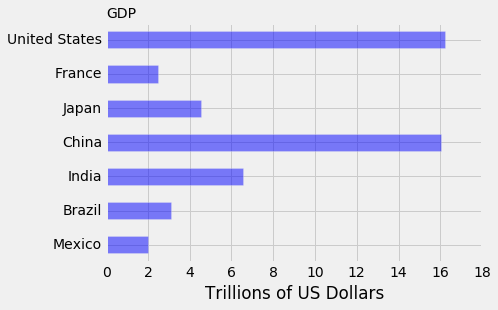Comment. For aficionados, the always tasteful xkcd style.

In :
plt.xkcd()
ax = df['gdp'].plot(kind='barh', alpha=0.5)
ax.set_title('GDP', loc='left', fontsize=14)
ax.set_xlabel('Trillions of US Dollars')
ax.set_ylabel('')

Out:
<matplotlib.text.Text at 0x7fdcd0a449e8>
/home/matthewmckay/anaconda/lib/python3.5/site-packages/matplotlib/font_manager.py:1287: UserWarning: findfont: Font family ['Humor Sans', 'Comic Sans MS'] not found. Falling back to Bitstream Vera Sans
(prop.get_family(), self.defaultFamily[fontext]))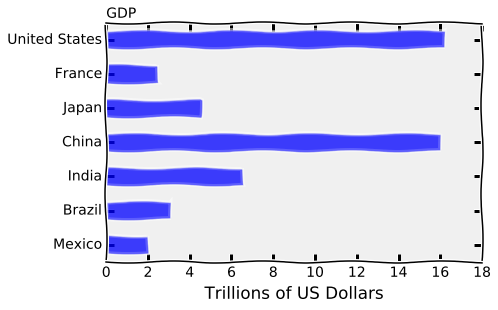Comment. We reset the style with these two lines:

In :
mpl.rcParams.update(mpl.rcParamsDefault)
%matplotlib inline


## Where does that leave us?¶

• We now have several ways to produce graphs.
• Next up: think about what we want to graph and why. The tools serve that higher purpose.
In [ ]: